# this keyword in Java: The Complete Guide

this keyword in Java language can be used inside a Method or constructor of the Class. It(this) works as the reference to a current Object, whose function or constructor is invoked. this keyword can refer to any member of a current object from within an instance method or a constructor.

## What is this keyword in Java?

‘this’ is the keyword in Java, which acts as a reference variable used to refer to the current object. ‘this’ can be used inside a constructor or a method.

Here is given the six usages of java this keyword.

1. This can be used to refer to the current class instance variable.
2. this keyword can be used to invoke the current class method (implicitly)
3. this() can be used to invoke a current class constructor.
4. this keyword can be passed as an argument in the method call.
5. this keyword can be passed as an argument in the constructor call.
6. this keyword can return the current class instance from the method.

## this keyword with a field(Instance Variable)

this keyword can be beneficial in the handling of Variable Hiding. We can not create the two instances/local variables with the same name. However, it is legal to create one instance variable & one local variable or Method parameter with the same name.

In this scenario, a local variable will hide an instance variable, called variable hiding in Java.

## Example of Variable Hiding

See the following code.

```class App {
int variable = 5;

public static void main(String args[]) {
App obj = new App();
obj.method(20);
obj.method();
}

void method(int variable) {
variable = 10;
System.out.println("Value of variable :" + variable);
}

void method() {
int variable = 40;
System.out.println("Value of variable :" + variable);
}
}```

See the output.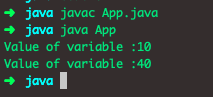## Ways to write ‘this’ keyword

1. this: It is used to call the class’s constructor.
2. this.data_member: It is used to call the data_member of the class.

## this keyword to call the constructor of the class

See the following program.

```class rectangle {
int length, breath;

rectangle(int l, int b) {
length = l;
breath = b;
}

rectangle(int len) {
this(len, len); /* calls the above constructor */
}

double area() {
return length * breath;
}
}

class Rect_Area {
public static void main(String[] er) {
rectangle r = new rectangle(6, 8);
System.out.println("The area of the rectangle is = " + r.area());
}
}```

See the output.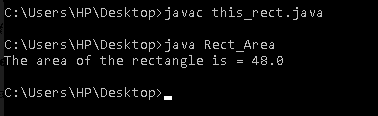#### this( ) keyword is also be chained the same as we chain constructor

See the following code.

```class cuboid {
double length , breath , height;
cuboid(int x){
length = x;
}
cuboid(int x, int y){
this(x);
breath = y;
}
cuboid(int x, int y, int z){
this(x, y);
height = z;
}
void show(){
System.out.println("Sides are : " + length + ", " + breath + ", " + height);
System.out.println("The area of cuboid is = "+(length*breath*height));
}
}
class This_cuboid {
public static void main(String[] er){
cuboid c1 = new cuboid(3,4,5);
c1.show();
}
}```

See the output.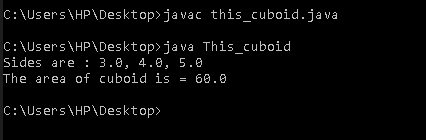## this.data_member

It is used to call the data_member of the current class.

### #Uses of this.data_member

1) ‘this’ keyword can be used to access the current object’s instance variable.

See the following program.

```class student {
String n;
int r;

student(String n, int r) {
this.n = n;
this.r = r;
}

double show() {
System.out.println(" Name= " + n + " Roll number = " + r);
return 0;
}

public static void main(String[] er) {
student s = new student("Shouvik", 20);
s.show();
}
}```

See the output.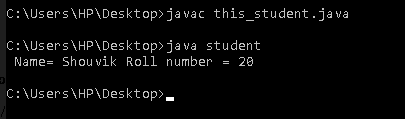2) this keyword can be used for invoking the current class method.

See the following program.

```class student {
void name() {
System.out.println(" My name is Shouvik");
}

void roll() {
System.out.println(" My roll number is 20 ");
this.name();
}
}

class this_method {
public static void main(String[] er) {
student s = new student();
s.roll();
}
}```

See the output.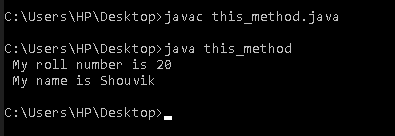3) this keyword can be used for invoking a current class constructor.

See the following program.

```class Run {
int r;

Run() /* First constructor */
{
this(10); // calling above constructor using this keyword.
}

Run(int a) /* Second constructor */
{
r = a;
}

public static void main(String[] er) {
Run ob = new Run(); /* creating the object */
System.out.println(ob.r);
}
}```

See the output.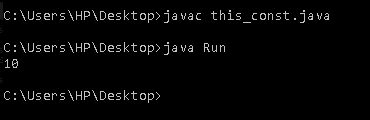4) We can use this keyword as an argument in the method call.

```class A {
void method_1(A obj) {
System.out.println("Class A method");
}

void method_2() {
method_1(this);
}

public static void main(String args[]) {
A a1 = new A();
a1.method_2();
}
}```

See the output.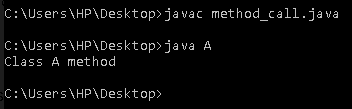5) We can use this keyword as an argument in the constructor call.

See the following program.

```class car {
D ob;

car(D ob) { // constructor
this.ob = ob;
}

void show() {
System.out.println(ob.value); // using data member of A4 class
}
}

class D {
int value = 50;

D() {
car c = new car(this);
c.show();
}

public static void main(String args[]) {
D a = new D();
}
}```

See the output.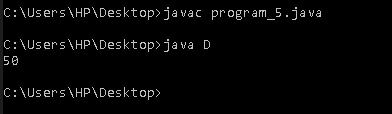6) this keyword is also used for returning the current instance of the class.

##### Syntax:
```return_type  method_name()
{
return this;
}```

See the following program.

```class school {
String name;
int roll;

school() {
name = "Shouvik";
roll = 20;
}

school get() {
return this;
}

void show() {
System.out.println("Name is " + name);
System.out.println("Roll number is= " + roll);
}

public static void main(String[] er) {
school sc = new school();
sc.get().show();
}
}```

See the output.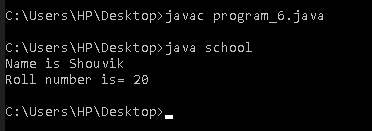#### Note:

We can’t use this( ) keyword and super keyword concurrently inside a constructor because both have to be the first call inside the constructor. So it is not possible to take this( ) and super( ) keyword inside a constructor.

When you assign  roll=20, that means the assignment is happing to the parameter while this.roll =20 means assignment happens to the instance variable.

So, There can be lots of usage of “this keyword”. For example, in the Java programming language, this is the reference variable that refers to a current object.

That’s it for this tutorial.

This site uses Akismet to reduce spam. Learn how your comment data is processed.